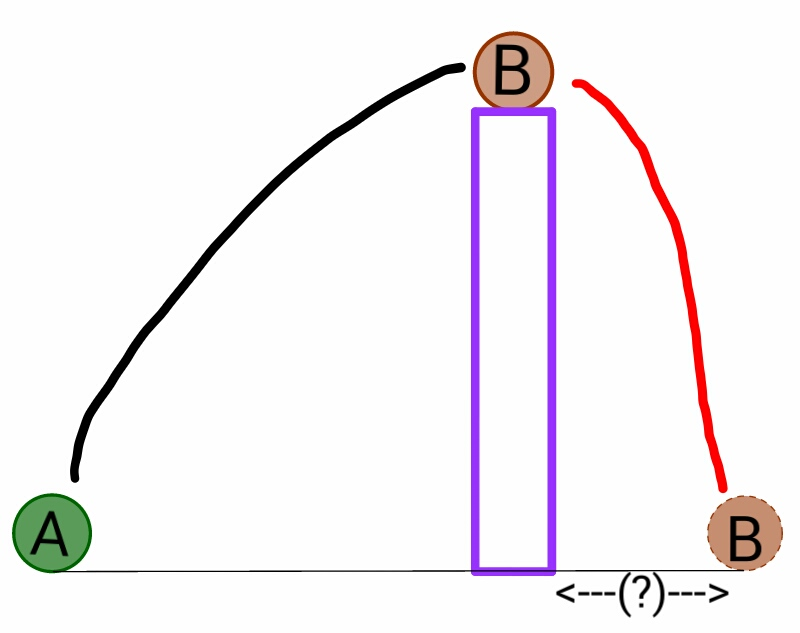# I won't possibly throw like that, would I?

Ball $A$ of mass $1\text{ kg}$ is thrown at an angle of ${45}^\circ$ with the horizontal with kinetic energy $50\text{ J}$ such that it hits ball $B$ of the same mass placed on the top of a pole. In this collision, half of the kinetic energy of ball $A$ at that instant is transferred to ball $B,$ causing ball $B$ to move in a forward direction. If it is known that the height at which ball $B$ was placed is the maximum height that the initial projectile of ball $A$ would have traveled, then find the distance of the final position of ball $B$ from the foot of the pole.

The figure below would help in understanding the situation:Details and Assumptions:

• Ball $B$ does not rebound after hitting the ground.
• Air friction is negligible.
• Take $g=10\text{ m/s}^2$ as the acceleration due to gravity.
• Give your answer (in $\text{m}$) to two decimal places.
×